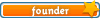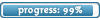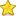Welcome

It is highly recommended that you have Javascript enabled; many features will not work unless you do. The recommended browsers are Firefox and Chrome; the board is also NOT mobile-friendly.

If you were referred by someone (ex. me, Jessica) please put their username in the referral box on the registration page. Ask them if you don't know their username.

If you are visiting for TESTING PURPOSES ONLY, this is the test account information:

Test

Select a forum to post in:

## Partial Fractions

Math topics

Moderators: Jessica, Teachers

Looking for a topic?
View the Math Topics Directory to find topics quicker under different kinds of math.

### Partial Fractions

Each fraction on the right side of the equation is a partial fraction, and together they make up the partial fraction decomposition on the left side.

Steps
1. Factor the Denominator.
2. Express factored form as a sum of denominators using A and B as numerators and set this equal to the original problem.
3. Eliminate the denominators by multiplying each side by the LCD.
4. Find the excluded values of x. (What would make the denominator equal 0?)
5. Substitute the excluded values into the equation and solve for A and B.
6. Substitute the values into step 2.

A.

x = −2

5 = −5B

B = −1

x = 3

10 = 5A

A = 2

B.

x = −4

4 = −2A

A = −2

x = −2

6 = 2B

B = 3

• 0Jessica
Board Owner
Topic AuthorSapphirePosts: 3,470
Topics: 1,244
Articles: 30
Joined: December 22nd, 2010, 8:04 pm